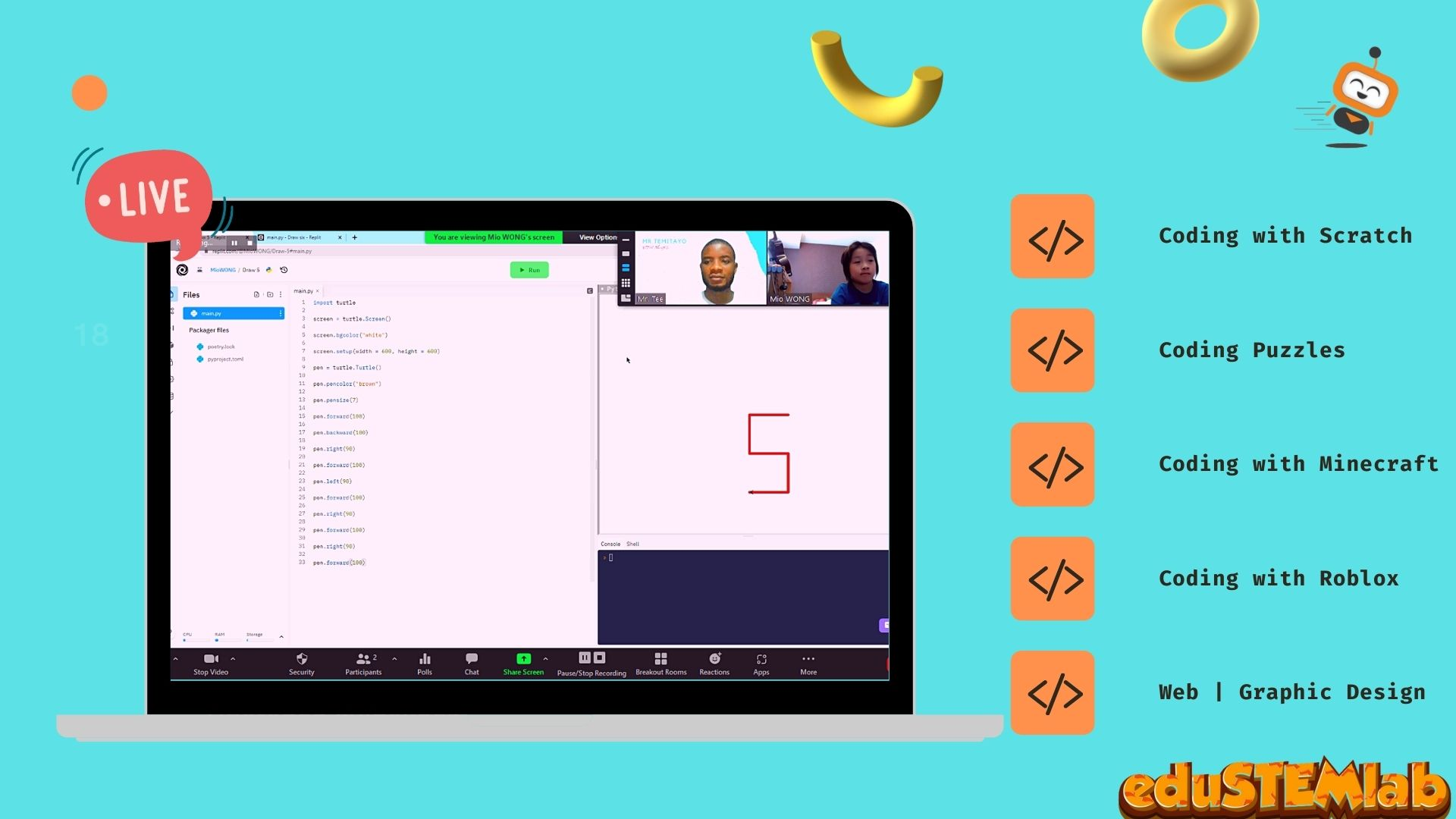# Be Globally Certified In Python

Python is a great text coding programming language for kids!

# Python

Python is a programming language that is particularly good for kids wanting to learn how to code. Python is widely used to teach students coding due to it`s easy to read and understand. Students can learn to write simple code to make their computer run a program.### What is an arithmetic operator:

An arithmetic operator is used with numeric values to perform common mathematical operations.

These operators are + (addition), – (subtraction), * (multiplication), / (division), and % (modulo)

Arithmetic operators perform their actions on numbers only

Below are examples of arithmetic operators

### How to use an arithmetic operator in python:

Alana scored 60 in English, 95 in Science, 100 in Mathematics and 75 in History. Create a python program to calculate her total score